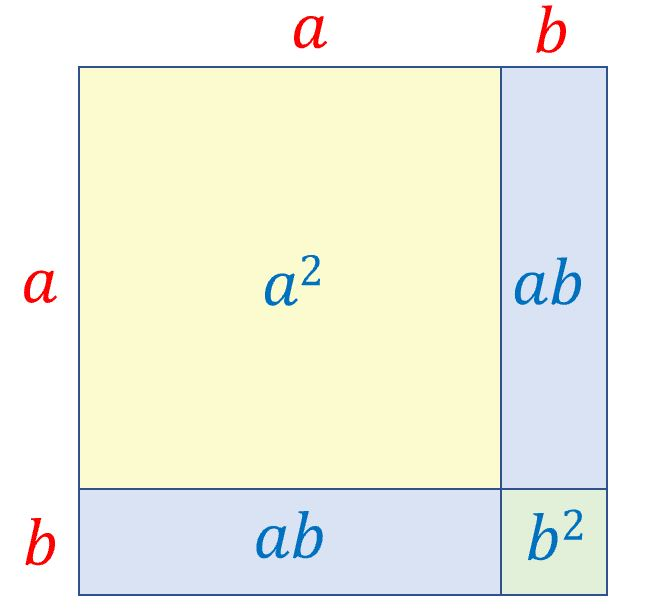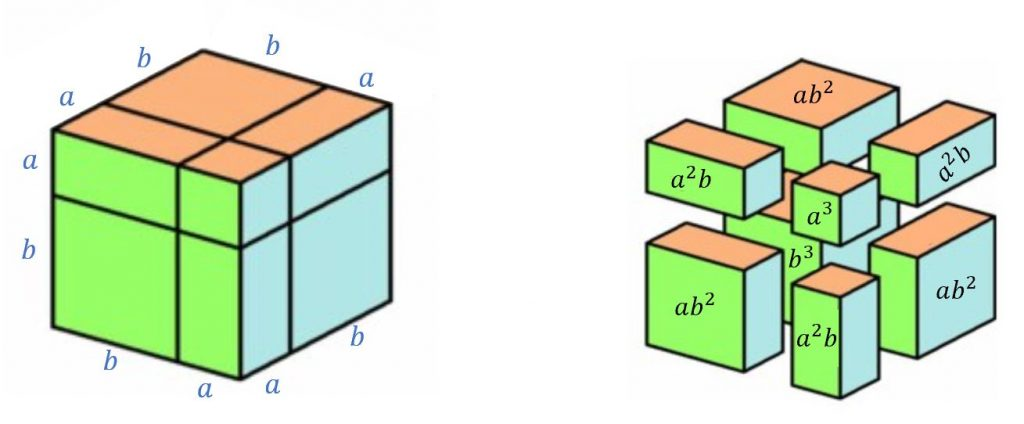# Pascal’s Triangle and its Secrets – Introduction

This is the first in a series of guest posts by David Benjamin, exploring the secrets of Pascal’s Triangle.

The triangle of Natural numbers below contains the first seven rows of what is called Pascal’s triangle. Each row begins and ends with the number 1, and each of the remaining numbers, from the third row onwards, is the sum of the two numbers ‘above’:

## History of Pascal’s Triangle

The triangle is named for Blaise Pascal – however, possibly the earliest discovery of the triangle was by the Islamic mathematician Abu Bekr ibn Muhammad ibn al-Husayn Al-Karaji who was born in Baghdad, Persia – now Iraq – in 953. His most important work was a treatise on algebra called Al-Fakhri.

One of Al-Karaji’s many other contributions to mathematics was proving that the sum of the first $n$ natural numbers was $\frac{1}{2}n(n+1)$, and discovering the wonderful identity

$(1 + 2 + 3 + \ldots + 10)^2 = 1^3 + 2^3 + 3^3 + … + 10^3$

Al-Karaji displayed this triangle of numbers in tabular form:

The Chinese mathematician Jia Xian (~1010-1070) produced his version approximately 500 years before Pascal was born. Jia Xian, also known as Chia Hsien, was a Palace eunuch of the Left Duty Group. Eunuchs were employed by the Emperor of China not only as guards of the women’s quarters to maintain their chastity but as advisors to the Emperor, often as Government ministers.

This Chinese triangle also appeared in 1303 in Jade Mirror of the Four Unknowns – the second book of Zhu Shijie, also known as Chu Shih-Chieh, who lived during the Yuan Dynasty (~1260-1320) and was one of the greatest Chinese Mathematicians, notable for his work on developing methods to deal with simultaneous equations.

Jia Xian wrote two books on mathematics: Huangdi Jiuzhang Suanjing Xicao [The Yellow Emperor’s detailed solutions to the Nine Chapters on the Mathematical Art  and Suanfa Xuegu Ji [A collection of ancient mathematical rules]. Both books are now lost but much of the content of Huangdi Jiuzhang Suanjing Xicao was revealed by the Chinese mathematician, Yang Hui (~1238-1298) in his book Xiangjie Jiuzhang Suanfa, published in 1261, in which he included details of Yang Hui’s triangle and the binomial coefficients.

## Binomials

A binomial is an algebraic expression which is the sum (or the difference) of two terms, say $(a + b)$. Jia Xian was able to expand $(a + b)^n$ from $n = 0$ to $n = 6$ and the coefficients of the expansion, displayed as rod numerals in Zhu Shijie’s book, are the numbers in the corresponding row of the triangle. Al-Karaji had expanded $(a + b)^3$ in Al-Fakhri and later $(a – b)^3$ and $(a + b)^4$.

Coefficients with value 1 are not used in algebraic expressions, and so $1a$ is written as $a$. Also $a^1$ and $b^1$ are written as $a$ and $b$ and $a^0$ and $b^0$ are both equal to 1.

The terms in each expansion involving powers of the variables a and b also follow a pattern.

$\begin{eqnarray*} (a + b)^0 &=& 1 \\ (a + b)^1 &=& a + b \\ (a + b)^2 &=& 1a^2b^0 + 2a^1b^1 + 1a^0b^2 = a^2 + 2ab + b^2\\ (a + b)^3 &=& 1a^3b^0 + 3a^2b^1 + 3a^1b^2 + 1a^0b^3 = a^3 + 3a^2b + 3ab^2 + b^3 \\ (a + b)^4 &=& a^4 + 4a^3b + 6a^2b^2 + 4ab^2 + b^4 \\ (a + b)^5 &=& a^5 + 5a^4b + 10a^3b^2 +10a^2b^3 + 5ab^4 + b^5 \\ (a + b)^6 &=& a^6 + 6a^5b + 15a^4b^2 + 20a^3b^3 + 15a^2b^4 + 6ab^5 + b^6 \end{eqnarray*}$

Below are geometric interpretations of the expansions of $(a+b)^2$ and $(a+b)^3$:$(a+b)^2$ represented geometrically$(a+b)^3$ represented geometrically

If we let $a=b=1$ in the expression of $(a+b)^n$, we can see that each row of Pascal’s triangle sums to a power of $2$, namely $2^n$ for $n=0,1,2,3,4,…$

If we let $a=1$ and $b=(-1)$ then each row of Pascal’s triangle sums to zero, but only for $n=1,2,3,4,…$ as $0^0$ is undefined. However the pattern of numbers in the triangle tease us to suggest that $0^0=1$…

## Enter Pascal

Blaise Pascal was born on the 19th June 1623 in Clermont (now Clermont-Ferrand), in the Auvergne region of France, and was recognised as a gifted mathematician by the age of 16. Blaise was one of four children. Their mother died when Blaise was only three years old, and in 1632 Etienne moved the family and settled in Paris.

Blaise died in 1662 at the relatively young age of 39, by which time he had made contributions to mathematics, hydraulics and philosophy.

In 1654 Pascal was corresponding with Pierre de Fermat about calculating the odds in a game of chance. This led Pascal to publish, in 1655, his Traité du triangle arithmétique, avec quelques autres petits traitez sur la mesme matière [Treatise on the arithmetic triangle, with some other small treatises on the same subject] – and the triangle of numbers in it became known as Pascal’s triangle. The correspondence between Pascal and Fermat was published in 1679 and that, along with the book, enhanced Pascal’s reputation as the founder of probability theory.

Hidden inside Pascal’s triangle are many wonderful patterns, connections and sequences with links to algebra and statistics. There are many avenues of delight, and future articles in this series will reveal some of the number sequences and geometric patterns hiding in the diagonals and rows of the triangle.

•#### David Benjamin

Teacher of mathematics [1975-2018], Author [Heinemann, Stanley Thornes], Key contributor teachitmaths, Mathematics software producer [Virtual Image]

### 7 Responses to “Pascal’s Triangle and its Secrets – Introduction”

1.Nicholas Mee

I really enjoyed the article. Very interesting and very well presented. I don’t remember ever seeing Al-Karaji’s identity before.

2.Anna
3.Anonymous